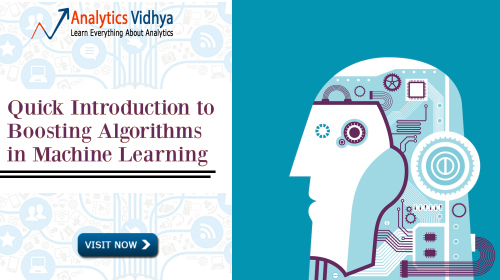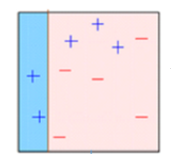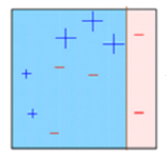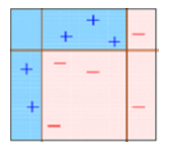Sunil Ray — Published On November 9, 2015 and Last Modified On August 25th, 2022

### Introduction

Lots of analyst misinterpret the term ‘boosting’ used in data science. Let me provide an interesting explanation of this term. Boosting grants power to machine learning models to improve their accuracy of prediction.

Boosting algorithms are one of the most widely used algorithm in data science competitions. The winners of our last hackathons agree that they try boosting algorithm to improve accuracy of their models.

In this article, I will explain how boosting algorithm works in very simple manner. I’ve also shared the Python codes below. I’ve skipped the intimidating mathematical derivations used in Boosting. Because, that wouldn’t have allowed me to explain this concept in simple terms.

Let’s get started.## What is Boosting?

Definition: The term ‘Boosting’ refers to a family of algorithms which converts weak learner to strong learners.

Let’s understand this definition in detail by solving a problem of spam email identification:

How would you classify an email as SPAM or not? Like everyone else, our initial approach would be to identify ‘spam’ and ‘not spam’ emails using following criteria. If:

1. Email has only one image file (promotional image), It’s a SPAM
2. Email has only link(s), It’s a SPAM
3. Email body consist of sentence like “You won a prize money of \$ xxxxxx”, It’s a SPAM
4. Email from our official domain “Analyticsvidhya.com” , Not a SPAM
5. Email from known source, Not a SPAM

Above, we’ve defined multiple rules to classify an email into ‘spam’ or ‘not spam’. But, do you think these rules individually are strong enough to successfully classify an email? No.

Individually, these rules are not powerful enough to classify an email into ‘spam’ or ‘not spam’. Therefore, these rules are called as weak learner.

To convert weak learner to strong learner, we’ll combine the prediction of each weak learner using methods like:
•   Using average/ weighted average
•   Considering prediction has higher vote

For example:  Above, we have defined 5 weak learners. Out of these 5, 3 are voted as ‘SPAM’ and 2 are voted as ‘Not a SPAM’. In this case, by default, we’ll consider an email as SPAM because we have higher(3) vote for ‘SPAM’.

## How Boosting Algorithms works?

Now we know that, boosting combines weak learner a.k.a. base learner to form a strong rule. An immediate question which should pop in your mind is, ‘How boosting identify weak rules?

To find weak rule, we apply base learning (ML) algorithms with a different distribution. Each time base learning algorithm is applied, it generates a new weak prediction rule. This is an iterative process. After many iterations, the boosting algorithm combines these weak rules into a single strong prediction rule.

Here’s another question which might haunt you, ‘How do we choose different distribution for each round?’

For choosing the right distribution, here are the following steps:

Step 1:  The base learner takes all the distributions and assign equal weight or attention to each observation.

Step 2: If there is any prediction error caused by first base learning algorithm, then we pay higher attention to observations having prediction error. Then, we apply the next base learning algorithm.

Step 3: Iterate Step 2 till the limit of base learning algorithm is reached or higher accuracy is achieved.

Finally, it combines the outputs from weak learner and creates  a strong learner which eventually improves the prediction power of the model. Boosting pays higher focus on examples which are mis-classiﬁed or have higher errors by preceding weak rules.

## Types of Boosting Algorithms

Underlying engine used for boosting algorithms can be anything.  It can be decision stamp, margin-maximizing classification algorithm etc. There are many boosting algorithms which use other types of engine such as:

3. XGBoost

In this article, we will focus on AdaBoost and Gradient Boosting followed by their respective python codes and will focus on XGboost in upcoming article.This diagram aptly explains Ada-boost. Let’s understand it closely:

Box 1: You can see that we have assigned equal weights to each data point and applied a decision stump to classify them as + (plus) or – (minus). The decision stump (D1) has generated vertical line at left side to classify the data points. We see that, this vertical line has incorrectly predicted three + (plus) as – (minus). In such case, we’ll assign higher weights to these three + (plus) and apply another decision stump.Box 2: Here, you can see that the size of three incorrectly predicted + (plus) is bigger as compared to rest of the data points. In this case, the second decision stump (D2) will try to predict them correctly. Now, a vertical line (D2) at right side of this box has classified three mis-classified + (plus) correctly. But again, it has caused mis-classification errors. This time with three -(minus). Again, we will assign higher weight to three – (minus) and apply another decision stump.Box 3: Here, three – (minus) are given higher weights. A decision stump (D3) is applied to predict these mis-classified observation correctly. This time a horizontal line is generated to classify + (plus) and – (minus) based on higher weight of mis-classified observation.Box 4: Here, we have combined D1, D2 and D3 to form a strong prediction having complex rule as compared to individual weak learner. You can see that this algorithm has classified these observation quite well as compared to any of individual weak learner.AdaBoost (Adaptive Boosting) : It works on similar method as discussed above. It fits a sequence of weak learners on different weighted training data. It starts by predicting original data set and gives equal weight to each observation. If prediction is incorrect using the first learner, then it gives higher weight to observation which have been predicted incorrectly. Being an iterative process, it continues to add learner(s) until a limit is reached in the number of models or accuracy.

Mostly, we use decision stamps with AdaBoost. But, we can use any machine learning algorithms as base learner if it accepts weight on training data set. We can use AdaBoost algorithms for both classification and regression problem.

You can refer article “Getting smart with Machine Learning – AdaBoost” to understand AdaBoost algorithms in more detail.

### Python Code

Here is a live coding window to get you started. You can run the codes and get the output in this window itself:

You can tune the parameters to optimize the performance of algorithms, I’ve mentioned below the key parameters for tuning:

• n_estimators: It controls the number of weak learners.
• learning_rate:Controls the contribution of weak learners in the final combination. There is a trade-off between learning_rate and n_estimators.
• base_estimators: It helps to specify different ML algorithm.

You can also tune the parameters of base learners to optimize its performance.

In gradient boosting, it trains many model sequentially. Each new model gradually minimizes the loss function (y = ax + b + e, e needs special attention as it is an error term) of the whole system using Gradient Descent method. The learning procedure consecutively fit new models to provide a more accurate estimate of the response variable.

The principle idea behind this algorithm is to construct new base learners which can be maximally correlated with negative gradient of the loss function, associated with the whole ensemble.  You can refer article “Learn Gradient Boosting Algorithm” to understand this concept using an example.

In Python Sklearn library, we use Gradient Tree Boosting or GBRT. It is a generalization of boosting to arbitrary differentiable loss functions. It can be used for both regression and classification problems.

### Python Code

```from sklearn.ensemble import GradientBoostingClassifier #For Classification
from sklearn.ensemble import GradientBoostingRegressor #For Regression```
```clf = GradientBoostingClassifier(n_estimators=100, learning_rate=1.0, max_depth=1)
clf.fit(X_train, y_train)```
• n_estimators: It controls the number of weak learners.
• learning_rate:Controls the contribution of weak learners in the final combination. There is a trade-off between learning_rate and n_estimators.
• max_depth: maximum depth of the individual regression estimators. The maximum depth limits the number of nodes in the tree. Tune this parameter for best performance; the best value depends on the interaction of the input variables.

You can tune loss function for better performance.

## End Note

In this article, we looked at boosting, one of the method of ensemble modeling to enhance the prediction power. Here, we have discussed the science behind boosting and its two types: AdaBoost and Gradient Boost. We also studied their respective python codes.

In my next article, I will discuss about another type of boosting algorithms which is now a days secret of wining data science competitions “XGBoost”.

### If you like what you just read & want to continue your analytics learning, subscribe to our emails, follow us on twitter or like our facebook page.###### Sunil Ray

I am a Business Analytics and Intelligence professional with deep experience in the Indian Insurance industry. I have worked for various multi-national Insurance companies in last 7 years.

### Our Top Authors

•